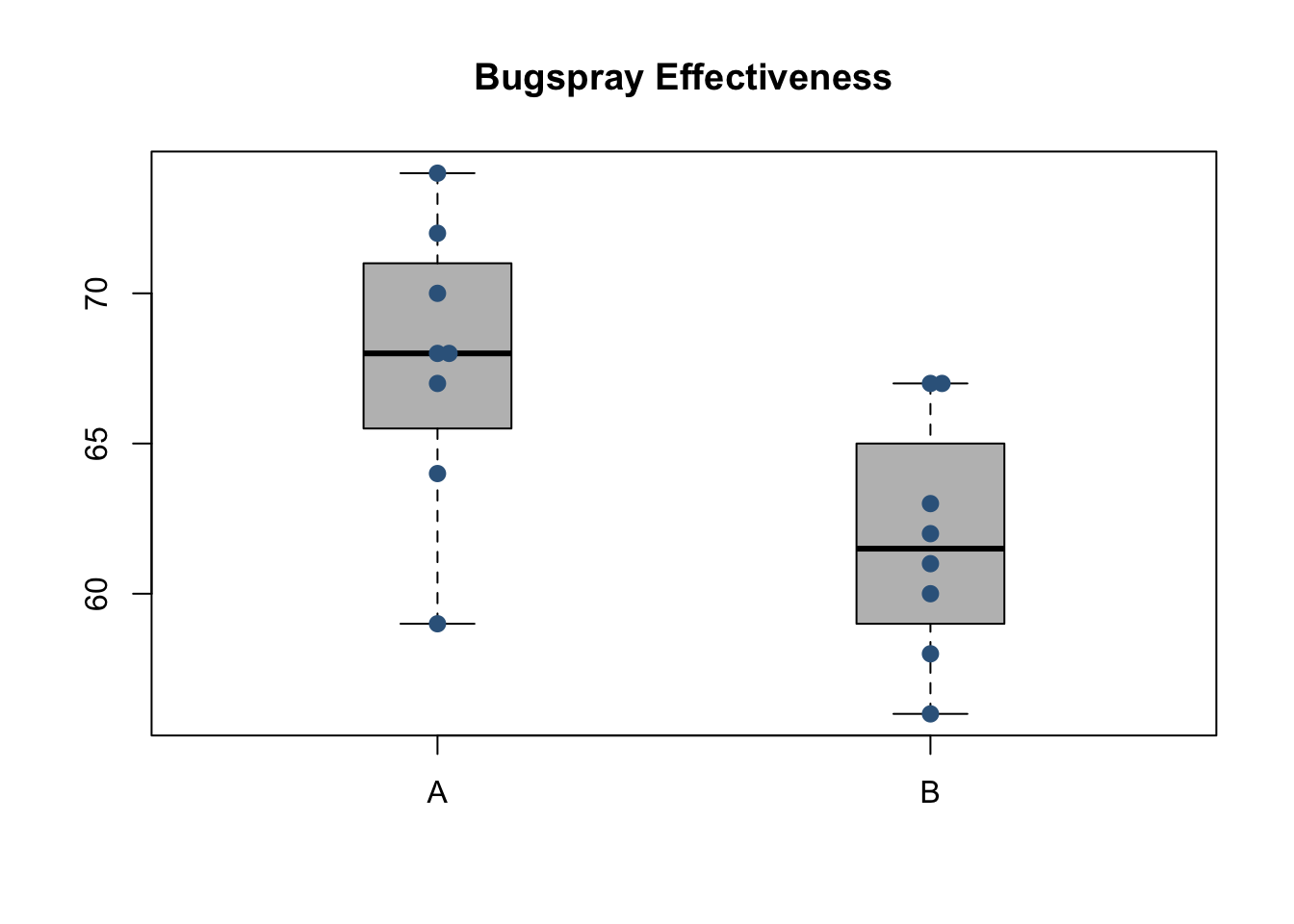#### Background

Note: the data for this example comes from the original 1945 paper Individual Comparison by Ranking Methods by Frank Wilcoxon.

The percent of flies (bugs) killed from two different concentrations of a certain spray were recorded from 16 different trials, 8 trials per treatment concentration. The experiment hypothesized that the center of the distributions of the percent killed by either concentration were the same. In other words, that both treatments were equally effective. The alternative hypothesis was that the treatments differed in their effectiveness. In short, we wish to know which bug spray is more effective at killing flies (bugs).

Spray Concentration Percent Killed
A 68, 68, 59, 72, 64, 67, 70, 74
B 60, 67, 61, 62, 67, 63, 56, 58

Formally, the null and alternative hypotheses are written as $H_0: \text{difference in medians} = 0$ $H_a: \text{difference in medians} \neq 0$

The significance level for this study will be set at $\alpha = 0.05$

#### Analysis

The side-by-side dotplots below (with boxplots overlaid) suggest that Spray A is more effective than Spray B at killing bugs.The actual summary values depicted in the plot above are shown in the following table.

.group min Q1 median Q3 max mean sd n missing
A 59 66.25 68.0 70.5 74 67.75 4.683 8 0
B 56 59.50 61.5 64.0 67 61.75 3.919 8 0

To determine if it is reasonable to infer that this conclusion is valid in general (for the full population) a Wilcoxon Rank Sum Test will be used. While the two samples are not identically shaped (shown in the boxplots above) they are not different enough to violate the assumption of identical shape and spread that is required by the Rank Sum Test.

Note that since there are ties present in the data, an exact $$p$$-value cannot be computed. An approximation will be used instead. Because of the small sample size, a continuity correction will also be applied. The results of the test are still valid and show sufficient evidence to reject the null hypothesis $$(p=0.01771 < \alpha)$$.


Wilcoxon rank sum test with continuity correction

data:  Killed by Spray
W = 55, p-value = 0.01771
alternative hypothesis: true location shift is not equal to 0

#### Interpretation

Spray A is the more effective spray. Sadly, it still only kills roughly 68% of bugs that make contact with the spray, on average.